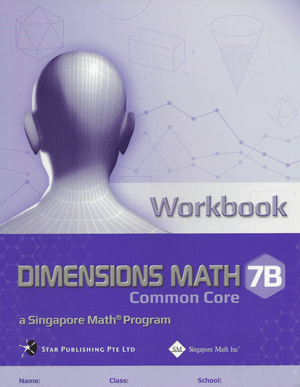# Singapore Math Dimensions Math Workbook 7B

Workbooks are the essential supplements to textbooks as they give students the opportunity to practice applying new concepts. Workbook exercises polish analytical skills and help students develop a stronger foundation in math through repetition and variation.

Features & Components:

• Basic Practice: Simple questions that drill comprehension of concepts.
• Further Practice: More difficult questions that involve direct application.
• Challenging Practice: Questions that require synthesis.
• Enrichment: Questions that demand higher order thinking, analysis, and reasoning.

The answer key at the back of the book provides answers only. Workbook Solutions (sold separately) contain fully worked solutions.

Note: Two workbooks (A and B) correspond to the two halves of the school year. Soft cover.

Dimensions Math® Workbook 7B

Chapter 9: Number Patterns

9.1 Number Patterns and Sequences
9.2 General Term of a Sequence

A. General Term
B. Application of Number Patterns

In A Nutshell

Chapter 10: Coordinates and Linear Graphs

10.1 Cartesian Coordinate System
10.2 Linear Graphs
10.3 Slopes of Linear Graphs

A. Positive Slopes
B. Negative Slopes
C. Special Cases

In A Nutshell

Chapter 11: Inequalities

11.1 Solving Simple Inequalities

A. Idea of Inequalities
B. Solving an Inequality

11.2 More Properties of Inequalities
11.3 Simple Linear Inequalities
11.4 Application of Simple Inequalities

In A Nutshell

Chapter 12: Perimeters and Areas of Plane Figures

12.1 Perimeters and Areas of a Square, a Rectangle, and a Triangle
12.2 Circumference and Area of a Circle
12.3 Area of a Parallelogram
12.4 Area of a Trapezoid
12.5 Perimeters and Areas of Composite Plane Figures

In A Nutshell

Chapter 13: Volumes and Surface Areas of Solids

13.1 Volumes and Total Surface Areas of a Cube and a Cuboid

A. Nets of Cube, Cuboid, and Other Prisms
B. Cube
C. Cuboid

13.2 Volume and Total Surface Area of a Prism

A. Prism
B. Volume of Prism
C. Surface Area of Prism

13.3 Volumes and Surface Areas of Composite Solids

A. Conversion between Different Units
B. Composite Solids

In A Nutshell

Chapter 14: Proportions

14.1 Scale Drawing
14.2 Map Scale and Calculation of Area

A. Map Scale
B. Calculation of Area

14.3 Direct Proportion
14.4 Inverse Proportion

In A Nutshell

Chapter 15: Data Handling

15.1 Collection of Data
15.2 Dot Plots
15.3 Measure of Center: Mean

A. Measure of Center
B. Mean
C. Using Mean to Compare Two Populations
D. Variation from the Mean

15.4 Measure of Center: Median
15.5 Mode

A. Definition of Mode
B. Comparison between Mean, Median, and Mode

In A Nutshell

Chapter 16: Probability of Simple Events

16.1 Set Notation

A. Sets
B. Equal Sets
C. Subsets
D. Universal Set and Empty Set
E. Complement of a Set

16.2 The Meaning of Probability

A. Introduction
B. Terms and Definitions

16.3 Sample Space

A. Sample Space
B. Basic Properties of Probabilities

In A Nutshell

Chapter 17: Probability of Combined Events

17.1 Probability of Simple Combined Events

A. Possibility Diagram
B. Tree Diagram

17.2 The Meaning of Probability
17.3 Independent Events
17.4 Further Probabilities

In A Nutshell